# Excel: If cell contains then count, sum, highlight, copy or delete

In our previous tutorial, we were looking at Excel If contains formulas that return some value to another column if a target cell contains a given value. Aside from that, what else can you do if a cell contains specific text or number? A variety of things such as counting or summing cells, highlighting, removing or copying entire rows, and more.

## Excel 'Count if cell contains' formula examples

In Microsoft Excel, there are two functions to count cells based on their values, COUNTIF and COUNTIFS. These functions cover most, though not all, scenarios. The below examples will teach you how to choose an appropriate Count if cell contains formula for your particular task.

### Count if cell contains any text

In situations when you want to count cells containing any text, use the asterisk wildcard character as the criteria in your COUNTIF formula:

COUNTIF(range,"*")

Or, use the SUMPRODUCT function in combination with ISTEXT:

SUMPRODUCT(--(ISTEX(range)))

In the second formula, the ISTEXT function evaluates each cell in the specified range and returns an array of TRUE (text) and FALSE (not text) values; the double unary operator (--) coerces TRUE and FALSE into 1's and 0's; and SUMPRODUCT adds up the numbers.

As shown in the screenshot below, both formulas yield the same result:

`=COUNTIF(A2:A10,"*")`

`=SUMPRODUCT(--(ISTEXT(A2:A10)))`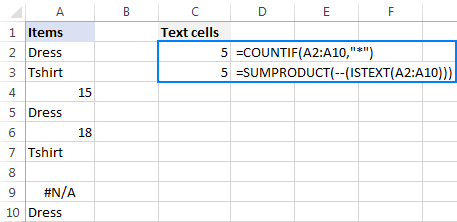You may also want to look at how to count non-empty cells in Excel.

### Count if cell contains specific text

To count cells that contain specific text, use a simple COUNTIF formula like shown below, where range is the cells to check and text is the text string to search for or a reference to the cell containing the text string.

COUNTIF(range,"text")

For example, to count cells in the range A2:A10 that contain the word "dress", use this formula:

`=COUNTIF(A2:A10, "dress")`

Or the one shown in the screenshot: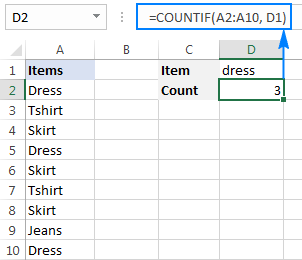You can find more formulas examples here: How to count cells with text in Excel: any, specific, filtered cells.

### Count if cell contains text (partial match)

To count cells that contain a certain substring, use the COUNTIF function with the asterisk wildcard character (*).

For example, to count how many cells in column A contain "dress" as part of their contents, use this formula:

`=COUNTIF(A2:A10,"*dress*")`

Or, type the desired text in some cell and concatenate that cell with the wildcard characters:

`=COUNTIF(A2:A10,"*"&D1&"*")`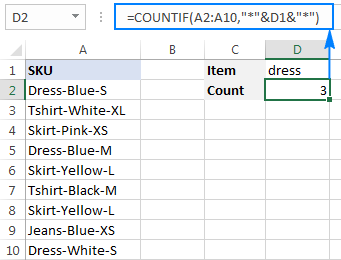### Count if cell contains multiple substrings (AND logic)

To count cells with multiple conditions, use the COUNTIFS function. Excel COUNTIFS can handle up to 127 range/criteria pairs, and only cells that meet all of the specified conditions will be counted.

For example, to find out how many cells in column A contain "dress" AND "blue", use one of the following formulas:

`=COUNTIFS(A2:A10,"*dress*", A2:A10,"*blue*")`

Or

`=COUNTIFS(A2:A10,"*"&D1&"*", A2:A10,"*"&D2&"*")`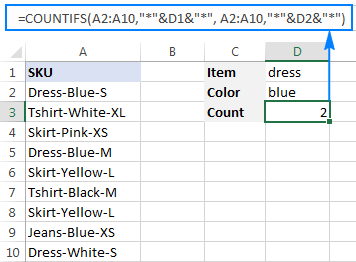### Count if cell contains number

The formula to count cells with numbers is the simplest formula one could imagine:

COUNT(range)

Please keep in mind that the COUNT function in Excel counts cells containing any numeric value including numbers, dates and times, because in terms of Excel the last two are also numbers.

In our case, the formula goes as follows:

`=COUNT(A2:A10)`

To count cells that DO NOT contain numbers, use the SUMPRODUCT function together with ISNUMBER and NOT:

`=SUMPRODUCT(--NOT(ISNUMBER(A2:A10)))`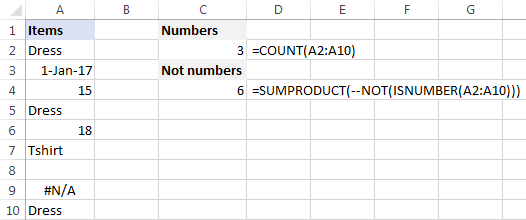For more examples, see Excel formulas to count cells with certain text.

## Sum if cell contains text

If you are looking for an Excel formula to find cells containing specific text and sum the corresponding values in another column, use the SUMIF function.

For example, to find out how many dresses are in stock, use this formula:

`=SUMIF(A2:A10,"*dress*",B2:B10)`

Where A2:A10 are the text values to check and B2:B10 are the numbers to sum.

Or, put the substring of interest in some cell (E1), and reference that cell in your formula, as shown in the screenshot below: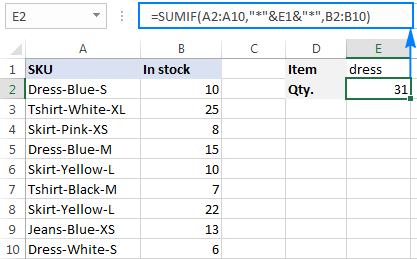To sum with multiple criteria, use the SUMIFS function.

For instance, to find out how many blue dresses are available, go with this formula:

`=SUMIFS(B2:B10, A2:A10,"*dress*",A2:A10,"*blue*")`

Or use this one:

`=SUMIFS(B2:B10, A2:A10,"*"&E1&"*",A2:A10,"*"&E2&"*")`

Where A2:A10 are the cells to check and B2:B10 are the cells to sum.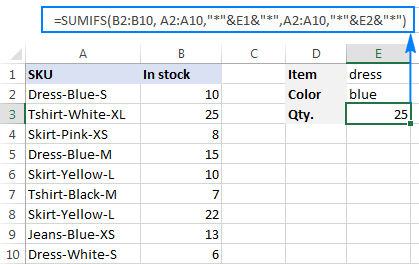## Perform different calculations based on cell value

In our last tutorial, we discussed three different formulas to test multiple conditions and return different values depending on the results of those tests. And now, let's see how you can perform different calculations depending on the value in a target cell.

Supposing you have sales numbers in column B and want to calculate bonuses based on those numbers: if a sale is over \$300, the bonus is 10%; for sales between \$201 and \$300 the bonus is 7%; for sales between \$101 and \$200 the bonus is 5%, and no bonus for under \$100 sales.

To have it done, simply multiply the sales (B2) by a corresponding percentage. How do you know which percentage to multiply by? By testing different conditions with nested IFs:

`=B2*IF(B2>=300,10%, IF(B2>=200,7%, IF(B2>=100,5%,0)))`

In real-life worksheets, it may be more convenient to input percentages in separate cells and reference those cells in your formula:

`=B2*IF(B2>=300,\$F\$5,IF(B2>=200,\$F\$4,IF(B2>=100,\$F\$3,\$F\$2)))`

The key thing is fixing the bonus cells' references with the \$ sign to prevent them from changing when you copy the formula down the column.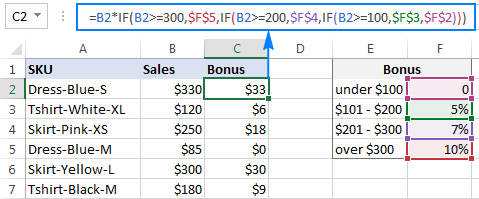## Excel conditional formatting if cell contains specific text

If you want to highlight cells with certain text, set up an Excel conditional formatting rule based on one of the following formulas.

### Case-insensitive:

SEARCH("text", topmost_cell)>0

### Case-sensitive:

FIND("text", topmost_cell)>0

For example, to highlight SKUs that contain the words "dress", make a conditional formatting rule with the below formula and apply it to as many cells in column A as you need beginning with cell A2:

`=SEARCH("dress", A2)>0`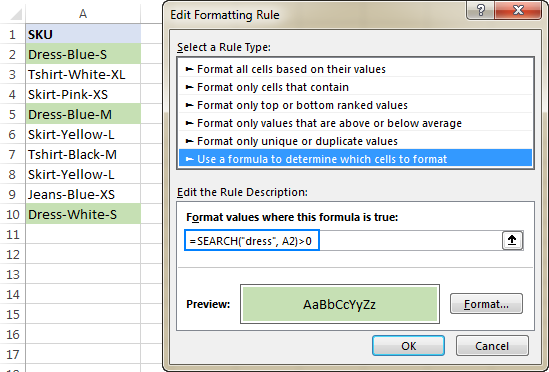### Excel conditional formatting formula: if cell contains text (multiple conditions)

To highlight cells that contain two or more text strings, nest several Search functions within an AND formula. For example, to highlight "blue dress" cells, create a rule based on this formula:

`=AND(SEARCH("dress", A2)>0, SEARCH("blue", A2)>0)`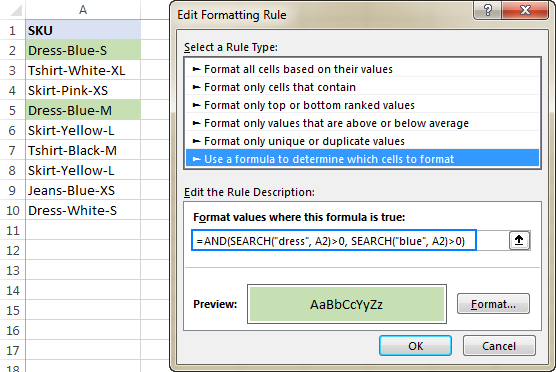For the detailed steps, please see How to create a conditional formatting rule with a formula.

## If cell contains certain text, remove entire row

In case you want to delete rows containing specific text, use Excel's Find and Replace feature in this way:

1. Select all cells you want to check.
2. Press Ctrl + F to open the Find and Replace dialog box.
3. In the Find what box, type the text or number you are looking for, and click the Find All
4. Click on any search result, and then press Ctrl + A to select all.
5. Click the Close button to close the Find and Replace
6. Press Ctrl and the minus button at the same time (Ctrl -), which is the Excel shortcut for Delete.
7. In the Delete dialog box, select Entire row, and click OK. Done!

In the screenshot below, we are deleting rows containing "dress":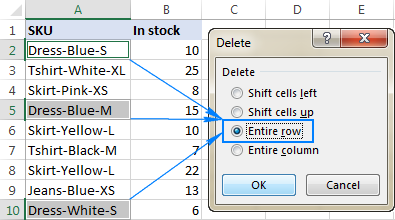## If cell contains, select or copy entire rows

In situations when you want to select or copy rows with relevant data, use Excel's AutoFilter to filter such rows. After that, press Ctrl + A to select the filtered data, Ctrl+C to copy it, and Ctrl+V to paste the data to another location.

To filter cells with two or more criteria, use Advanced Filter to find such cells, and then copy the entire rows with the results or extract only specific columns.

This is how you manipulate cells based on their value in Excel. I thank you for reading and hope to see you on our blog next week!

## Practice workbook

Excel If Cell Contains Then - examples (.xlsx file)

## You may also be interested in

1. I want a formula where the cells in a specific range will be counted based on data and criteria in another range.
in the other hand how many cell is in A1:A10 if there is Bi=10,i=1 to 1o

2. Thanl you for the response. That equation doesn't take in to account the other sheet that has all the data to pull from. What is the best way for me to send you the data?

3. I have two sets of data side by side, each with a country and a value. If the country differs (ie text A is different to text B), I want the value of the second country.

So essentially what is the formula within sumif to say 'if text A does not equal text B, then ?
Help!

• Hi! If I understand your task correctly, the following tutorial should help: Compare two columns for matches and differences. If you want to compare the values in the corresponding rows, try the IF formula:

=IF(A1=D1,B1,E1)

I hope it’ll be helpful. If this is not what you wanted, please describe the problem in more detail. Give an example of the source data and the expected result.

4. Hi there,
I have columns
A B C D E F G H I
Pay# Milestone Date Amount Paid On Pay# Milestone Date Amount Paid On TOTAL PAID
1 2023-01-23 5000 2023-02-18 2 2023-10-03 3000

I would like to know how can I create a formula to add the AMOUNTS from column c and g in the TOTAL PAID column (column I) just if the payment has a date in the PAID ON cells .

Thank you

• Hi! I hope you have studied the recommendations in the tutorial above. It contains answers to your question. Try this formula in column I:

=IF(ISNUMBER(H2),G2+C2,0)

5. Hello,

I'm hoping you can help me - I would like an Excel formula to calculate the following for Gain/Loss:

If cell F is greater than cell C, the answer in cell G is _______, but if cell C is greater than cell F, the answer in cell G is _________.

Example:
A B C D E F G H
Quantity Bought Buy price Total Buy \$ Quantity Sold Sell Price Total Sell \$ Gain / Loss Running Gain/Loss
100 \$106.22 \$10,622.00 100 \$112.52 \$11,252.00 \$ 630.00 \$630.00
100 \$ 89.34 \$ 8,934.00 100 \$ 78.56 \$ 7,856.00 -\$1,078.00 -\$448.00

Thank-you!
Kasey

6. I want to find the \$\$ sold of each pattern in a list of products. Column B contains the description, a string of characters that also contains a pattern name. Column E contains the \$\$ sold of each product. In Column F I have listed each pattern name, and I would like Column G to calculate the total \$\$ sold in each pattern. I just can't figure out how to do this.

• Hi! You can find the sum by condition using the SUMIF function. If there are several conditions, use the SUMIFS or SUMPRODUCT function. Without having an example of your data, it is impossible to recommend you an exact formula.

• Thanks for answering, here's a sample of the data:

Col A (description):
Bee Cheese Knife
Bottle Opener Stag
Mini Pheasant Stopper
Antler Opener
Coasters Antler
Bee Beer Glass
Stag Placemat
2 Round Stag Glasses

Col B (qty sold)
12
6
9
18
7
8
12
10

Col C (\$ sold)
\$375
\$127
\$3987
\$20
\$987
\$324
\$777
\$987

Then I have the pattern name list:
Bee
Stag
Pheasant
Antler

Next to each pattern name, I want to sum the qty and the \$ , so for example, the output For "Bee" would be qty 20, \$699.

• Hi! If I understand your task correctly, the following formula should work for you:

=SUMPRODUCT(ISNUMBER(SEARCH("Bee",A2:A20))*C2:C20)

• That works perfectly, thank you so much!

7. I need a formula that looks at Cell A1, which contains Account number then finds the same Account number in A1:A200 and then gives me the corresponding data from B1:B200

8. I am looking to calculate from a spreadsheet, a win-loss record for a team based on the day of the week. If cells a2-a100 represent the day of the week, and cells f2-f100 represent win or loss, how would i write the formula to calculate the winning percentage of all games played on saturday?

9. Hi,
I need to work out how to Sum values when they are coded in a different column e.g.:

Column A has codes:
4201
4605
4807
4201
4598
4605
4201

Column B has £ values against those codes.

I need to find a way to sum the values in column B each time 4201 (for example) occurs in Column A without having to add it up each time. Is there a way to do this?

• Hi! You can use the SUMIF function to calculate the sum of the values by condition. For example,

=SUMIF(A2:A20,4201,B2:B20)

10. Having trouble figuring out a solution. I have two (2) columns with a number of rows (likely a few more than shown below). I need to find text/data matches in column A (ENTRANT), add the respective POINTS from the same row(s) in column B together, and then produce a top five (5) in POINTS where the ENTRANT name and total POINTS are provided by the function/calculation. I'm fine if the results use all ENTRANT names in the Table but I only truly need the top five (5). But I need the results to be in order by POINTS with the highest number at the top. The ENTRANT names will change every so often so I cannot have the function etc searching for a specific name; the names have to be located and matched up (if that makes sense). Also, if POINTS totals are equal, then I don't really care if the names are then alphabetical (but it wouldn't hurt); I'm good either way.

Sample data in [A1:B15] with HEADER ROW in Table.

ENTRANT POINTS
Tyler 5
David 5
Zac 3
Josh 3
Tyler 5
Mark 5
Andy 3
Dale 3
Tim 15
Karl 10
Jacob 5
Tyler 15
Dale 10
Jacob 5

The results should display similar to the following:

Tyler 25
Tim 15
Dale 13
Karl 10
Jacob 8

• Hello!
To get a list that is sorted by points, you can get a list of names using the UNUQUE function, calculate the sum of points for each name using the COUNTIF function, and then combine these values into one array using the HSTACK function:

=SORT(HSTACK(UNIQUE(\$A\$1:\$A\$14),SUMIF(\$A\$1:\$A\$14,UNIQUE(\$A\$1:\$A\$14),\$B\$1:\$B\$14)),2,-1)

To extract the first 5 rows from this array, use TAKE function :

=TAKE(SORT(HSTACK(UNIQUE(\$A\$1:\$A\$14),SUMIF(\$A\$1:\$A\$14,UNIQUE(\$A\$1:\$A\$14),\$B\$1:\$B\$14)),2,-1),5)

11. I have a small set of non-linear tabular data that spans vertically and horizontally. Rows are actually based on dates of a month while the columns do not have any kind of headings. Each entry occupies two cells in a row e.g. [A1:date][B1:amount][C1:label][D1:amount][E1:label]. I wanted to sum up amounts in the cells that contain a certain label in the corresponding cell. SUMIFS seemed to be a good function but it only allows multiple ranges for criteria but not for the sum ranges.

Sample data in [A1:G2]

1-May-23 | 300 | ABC | 200 | DEF | 100 | XYZ
2-May-23 | 100 | EDX | 400 | GHL | 100 | ABC

Problem: SUM amounts that have ABC in corresponding cells.

Thanks

• Hi! You can also use the SUMPRODUCT function to find the sum or quantity of values for multiple criteria.

=SUMPRODUCT((B1:B10)*(C1:C10="ABC")+(D1:D10)*(E1:E10="ABC")+(F1:F10)*(G1:G10="ABC"))

The following tutorial should help: Excel SUMPRODUCT function with multiple criteria.

12. Hello,

I have data in column A that has a variety of different variables (ABC, GHY, DUY, IKL, ABC, ABC, DUY, LJK, ABC, IOP, ABC). I have varied dollar amounts in column D that correspond with column A. I want to add all the dollar amounts that are associated with ABC. I have tried but cannot figure this out.

13. I have a spreadsheet laid out like this. I have been summing the batch total by manually selecting the amount with that batch number, but is there a function to use? Sometimes amounts in the same batch are dozens of lines down, and this becomes tedious.

Amount Batch Number Batch Total
35.00 10657 77.00
36.00 11204
71.00

42.00 10657 91.00
55.00 11204
97.00

14. Hello. I have a template that I am trying to build to track invoices for clients. For example, I want the template to be able to have an input tab where I put Dr Smith in column A and in column B \$700 (who the bill is from and how much they spent) there will be multiple entries for each of my clients. I want to create a summary page that will look at the input tab and be able to list out all the bills (Dr Smith, Dr Marvin, General Hospital etc.) and the total amount they spent at each place. There will be multiple entries for each place that they spend money at, so there may be 5 entries for Walmart and 7 entries for Target. I want to create an itemized list on my input tab and on my summary tab have a formula that will populate the names and sum the amounts. I am not sure if this can be done. Any help would be greatly appreciated.

INPUT TAB WOULD LOOK LIKE THIS

DR SMITH \$700
DR KATZ \$500
DR KATZ \$350
DR DOE \$200
DR KATZ \$250
DR KING \$300
VA HOSPITAL \$100
VA HOSPITAL \$250

SUMMARY TAB WOULD LOOK LIKE THIS

Dr Smith \$700
Dr Katz \$1,100
Dr Doe \$200
Dr King \$300
VA Hospital \$350

15. I would appreciate any help
I have a spreadsheet with scores from events. Each person has participated in four events and they have four separated scores. I have assigned a point system for scores (i.e a 9 would give someone 20 points). is there a formula(function) that can look at the four cells of scores, determine if they qualify for points assigned and sum them into one cell of total points?

16. I have read ur example in "Perform different calculations based on cell value". Is there another simple way formula without using IF?
Because i have many tier in "Bonus". it too long if using IF

17. Hello,

On our employee vacation tracker, I'm trying to add up any cells with a "V" value over multiple sheets. When I used the =COUNTIF(January!C7:AG7,"*V*") it works for that sheet, but I'm not sure what the formula is to add all the sheets for January to December for each employee. I've tried this =COUNTIF(January:December!C7:AG7,"V") but I get an error. Thanks in advance for your help!

18. I have a table with data validation for the payment frequency to keep the spelling consistent.

I have table columns: Category, Payment Frequency (look up column), Payment Amount, Monthly Amount (cell multiplied or divided in formula)
Calculation: When I enter the payment frequency and the payment amount, I want to calculate the monthly payment amount.

Payment Frequency is calculated: monthly (*1), yearly (/12), bimonthly (*6), biweekly (*26/12), quarterly (*4/12), One time payment (*0) [I am not sure how to make that fit with the other payment options...]

Thanks :)

• * Sorry, thought I had that clearer. The Monthly Amount is the cell in which I will insert the formula. The payment amount is the one multiplied/divided based on the payment frequency. The multiplier/divisor is in the brackets after the given payment frequency.

19. I am trying to tally attendance at programs based on age groups and type of program. I'm having issues getting a 0 total of the Sum of 3 age groups if type column contains "X" text. Using similar =SUMIF(D2:D6,"On",A2:C6) I get 23 to display in formula's box, but for =SUMIF(D2:D6,"Off",A2:C6) I get zero instead of 15? Please help me find & fix my error.
A B C D
R1 Juv Teen Adult On/Off Site
R2 5 0 0 On
R3 0 7 6 On
R4 0 0 3 Off
R5 10 0 2 Off
R6 0 4 1 On

On Site total = 23
Off Site total = 15

20. Hello,

I'm stumped on auto populating a cell. What I want to input is if sheet 2, column A contains any of the same text as sheet 1, column A. Then sheet 2 column 3 will auto populate the same numbers that are showing on Sheet 1, column 3.

21. HI, Im looking for help with formatting a cell. So I want cell \$G\$277 to show as cell multiplied by .9789 if cell \$E\$277 shows as "bio". could anyone tell me how to input this please? thanks

• sorry i wrote this incorrectly i mean to type

HI, Im looking for help with formatting a cell. So I want cell \$G\$277 to show as cell \$H\$277 multiplied by .9789 if cell \$E\$277 shows as "bio". could anyone tell me how to input this please? thanks

• Hi!
With formatting, you cannot change the value of a cell. To change the value of a cell by condition, use the IF function.

• Hi, thanks for the reply. I know i cant change the value of a cell, im trying to populate a cell based on an equation/formula based on a result from another cell but i just dont know how to write the equation.

I want for instance cell A1 to show the result of cell B1 multiplied by 0.9789 as the "true" if cell C1 has the word "Bio" in it, does that make sense? thanks

• Hi!
Cell A1 can contain either a value or a formula like this:

=IF(C1="bio",B1*0.9789,B1)

• Hi, I've tried that but nothing happens in the cell even though C1 has the word in it and B1 has a number populated in it ready for the formula to make the result in cell A1

thanks

• I need to do this through conditional formatting as there is other information inputted that i need it to ignore

thanks

22. i have this formula in a column =IF(COUNTIFS(\$I:\$I,I2,\$Z:\$Z,"YES"),"OK","NO") the "YES" result corresponds to another value as a result of look up on the other sheet. i would like to populate that lookup value on the entire next column.

• Hi!
Sorry, it's not quite clear what you are trying to achieve. Could you please describe it in more detail?

• hi sorry for that.. here in my table below, i have two groupings. In the column C is where my formula as =IF(COUNTIFS(\$A:\$A,A2,\$C:\$C,"YES"),"OK","NO"). All the YES value in Column C ( as Apple and Milk ) is from my table in the other sheet with corresponding value of Fruits and Drinks. I need to put all the lookup result in the all cell for each group in Column E. how can i do it.

Column A Column B Column C Column D Column E
IMS Cherry No Ok Fruits
IMS Apple Yes Ok Fruits
IMS Soda No Ok Fruits
IMS2 Soda No Ok Drinks
IMS2 Milk Yes Ok Drinks
IMS2 Orange No Ok Drinks

• Hi!
Your explanations are still not clear. I assume that your formula is not in C but in column D. This formula determines if there are duplicate rows. The results of this formula do not match with your data.
Explain "lookup result" - what are you looking for and where.
As it's currently written, it's hard to tell exactly what you're asking.

• Hi!

Apologies.. yes my formula is n Column D where it becomes "OK" as it found "Yes" in Column C.

First is , i have to vlookup the DATA in Column B from the other sheet so i can have the YES or NO in Column C ( like the APPLE and Milk as YES value in Column C )

Second, in Column D is where i have the IF formula whether "OK" or "NO" .

Third, in Column E, something i need to get done. The YES value in Column C ( as APPLE and MILK corresponds to Fruit and Drink from other Sheet (so vlookup Apple will give me a result value of Fruit.)

so here in Column E i need to have a condition that if after the vlookup, the result value ( as Fruit ) will be filled in the certain cell per Group ( 3 cell per Column A groupings as per my sample.)

my apologies if i cant still explain it clearly here.. many thanks!

• Hi!
To check data from column B on another sheet, you can use the MATCH function like this:

=IF(ISNUMBER(MATCH(Sheet1!B1,Sheet2!B1:B100,0)),"Yes","No")

I don't understand what you want to do in column E.

• Thank you ..

i can check the data from column B on another sheet by the this formula

=IF(ISNA(INDEX(Sheet2!B1:B2,MATCH(TRUE,INDEX(Sheet2!A1:A2='Sheet1'!B1,0),0))),"YES","NO")

sheet2:
Column A Column B
Apple Fruits
Milk Drinks

so in Sheet1: Column E
What i want in Column E is to fill the cell with "Fruits" without the #N/A

and this is what i am having now in Column E (based on my table above)

Column E
#N/A
Fruits
#N/A
#N/A
Drinks

instead i want to have the formula which will gives me this.

Column E
Fruits
Fruits
Fruits
Drinks
Drinks
Drinks

23. Need help writing a formula to calculate percentage of invoices validated with payment dates. Essentially, we want to write a formula to generate a count of the cells with dates in them, and to exclude the cells with nothing. Based off this, we are building a gauge chart to depict the percentage of invoices validated. Min value would = 0 and Max value would = total invoices (with & without dates) and this formula would be the main data point showing percentage of invoices ONLY with payment dates tied to them.

• Hello!
To count the number of cells with dates, try this instruction: Count if blank or not blank. I hope it’ll be helpful. If this is not what you wanted, please describe the problem in more detail.

24. I'm looking for a formula that counts the number of cells that contain ANY text, and sum the number of cells in a cell below.
I need to sumerize how many clients each day, and obviously they all have diffrent names, and its always changing. As a bouns I'd like to enter that sum into another spreadsheet, on a specific days cell. the 2nd part is not vital. I'm running a homeless shelter and finding this formula stuff hard to absorb, lol.

• So I figured the first part out, and feel free to ignore the second. I'm trying to learn everything and have a couple course type files, and now found this jem. Thanks for helping people out so much, very kind of you all.

• Hi!
Pay attention to the first paragraph of the article above.

25. Hi.

Is possible to sum all WA11?

WA11 4
AdBlue 3, WA11 3, shift 4

... and everything is in one column

26. I have a time sheet that has a job code that I would like excel to reference to add up the hours that each employee has worked for that job to come up with the total hours worked on that job for the week.

So, if there is a letter in one cell, I want excel to take the numbers from another cell and add them all together for every occurrence of that letter in the table.

• I'm not sure that is the correct formula.

Jobsite Code Job Total
AB A
CD C
FG D

Employee Mon Job Tue Job Wed Job
John 6 A 8 C 7 D
Joe 9 C 7 A 8 C

Taking the table above, I want Excel to look for A and add the hours from the corresponding cell; so, there's an A in D7 and F8, I want Excel to add the hours in D6 (6 hours) and F7 (7) together for total hours worked on job A

• Hi!
You can use SUMIF formula:

=SUMIF(C1:C10,"A",B1:B10)+SUMIF(E1:E10,"A",D1:D10)

Or SUM formula:

=SUM((B1:B10)*(--(C1:C10="A")),(D1:D10)*(--(E1:E10="A")))

27. What formula would I use (or is there one) to total the hours employees took to take certain staff training requirements (example table below):

A B C D E F G
1 Employee Name Course 1 Course 2 Course 3 Course 4 Course 5 Hours Completed
2 Course Length 3.25 1.25 1.50 1.00 0.75
3 Employee 1 10/17/22 10/12/22 10/12/22
4 Employee 2 10/16/22 10/04/22 10/12/22
5 Employee 3 10/17/22 10/13/22 10/13/22
6 Employee 4 10/17/22 10/17/22 10/11/22 10/11/22

• Hi!
Based on your description, it is hard to completely understand your task. However, I’ll try to guess and offer you use SUMPRODUCT function.

=SUMPRODUCT(B1:F1,--(B2:F2<>""))

• Sorry the table didn't come across very well, but that's exactly the formula I needed. Thank you!

28. Is possible to sum this text?

Some text in cell. r=11

R=1,5. Some text in cell.

Sum all r=? and, that's in one row.

29. Hello.

• Thank you very much

30. Hi i want to add following numbers which are present in a row

23,45,#N/A, 56,#N/A, 756,...
Please suggest the formula to solve it.

• I've got the same issue I think Baiju is having, in that Excel isn't clear on how you add up a series of columns or rows if some of the cells in the range have #NA or #NUM instead of a number.

I've got someone's spreadsheet I'm trying to salvage. They're trying to track the total invoices they process each month, amount of days to process each one, the number that are late (>30 days), and the \$ amount for the invoices that are late. So, I've been using =DATEDIF and =IF(AND and =COUNTIF formulas to take a stab.

It's cumbersome but would be working, except their spreadsheet includes all their invoices, including those that haven't cleared yet. As a result I get #NUM! or #NA in those cells. When I try to sum the monetary values for the month (either =SUM or =COUNTIF) it fails because of the #NUM and #NA in the range. I haven't been able to find an explanation on what to do when you've got non-numbers in a column you're trying to add, so any advice you can provide would be appreciated. Thanks.

• Hello!
Use the IFERROR function in formulas to avoid getting an error message. To calculate the sum, you can use the AGGEGATE function instead of the SUM function. If the second argument to this function is 6, then errors will be ignored.

31. Hi,

I need help to assign weights to text. For example, in the following response table,

Question No Response Weights
Q1 Red 1
Q2 Blue 2
Q3 Green 1
Q4 Red 2

Clearly, questions 2 and 4 are having higher importance. When commuting the response, it needs to read as
Red: (Q1*1+Q4*2) = 3
Blue: (Q2*2) =2
Green: (Q3*1) =1
Total =7 (not 4)

32. I'm trying to make a formula in which if an exact value is can get 4 different values in 4 different cells . Eg if A1=4 then B2=12, C2=199, D2 =122,E2=78

• Hi!
We wrote many times that an Excel formula can change the value only in the cell in which it is written. You need to use a VBA macro.
Or use an IF formula in each cell.

33. Hi and thank you.

Stuck trying to work out formula for staff roster, whereby in a row of cells x 7 (one week) each shift worked (x 3 shifts, which equal 12 hours each) adds the sum of these shifts to a separate cell.
Eg.. Staff Name / Sum of Total Hrs / LD / / LD / LD
Should look like-
Staff name / 36 / LD / / LD / LD

This will actually be over 6- week roster, but just trying to get an idea of what to do in the above eg.
Thanks

• Hi!
I’m not sure I got you right since the description you provided is not entirely clear. However, it seems to me that the formula below will work for you:

=COUNTIF(C1:I1,"LD")*12

Use the COUNTIF function to count the number of specific values in seven cells.

34. Hello,

I'd really appreciate help with a formula.

Column E is an IF statement and depending on a date range puts "YES" or "NO" in column E.

I'm trying to get a separate cell to provide the SUM of the number of "YES"s. SUM and SUMIF do not appear to work. For example, I have attempted the formula =SUMIF(E2:E20,"YES"). It incorrectly provides "0" as the answer. Is this because Column E is a separate formula itself. How do I get around this?

• Hello!
The SUMIF formula works with the results of formulas in the same way as with normal values. Your formula should work. Check what values are returned by your formulas in cells E2:E20. Perhaps there are extra spaces.

35. Hello,

I would appreciate your help on a formula.

I am creating a vertical employee absence calendar in Excel (dates at the top, names on the side) with leave entered as text: holiday (H), half day holiday (HH), sick leave (S), and half day sick leave (SH).

I would like to add a row total for each person.

With COUNTA all entries = 1
So for e.g. H + S + HH should add up to 2.5 but COUNTA shows 3.

How can Excel add up different values for each type of text? So that that H = 1, HH = 0.5, S=1 and SH=0.5?

Valerie

• Hi!
The COUNTA function counts the number of cells. So it cannot return a fractional number. Replace letters with numbers using the IF function:

=IF(A1="H",1,0)+IF(A2="HH",0.5,0)

• Hi there. I am trying to write a formula where I need to count how much three people spent.
Name cost. Name total spent
Me 300 me. ?
You 150 you. ?
Her 200 Her. ?
Me 140
You 200
Her 500

• Hi! I am not sure I fully understand what you mean.

• I have to calculate a total cost per date range for each event. I have a sheet that has the daily rate for different pay scales. I am trying to get the total cost for example: Cell B8 has a drop down for different pay grades. Cell E8 has the start date. Cell F8 has the end date. Sheet 2 has the data to pull from. Sheet 2 column B has the pay grades. Column C has the daily pay rate. How do I get on Sheet 1 Cell H8 to calculate that?

• Hi! I need to see your data to give you an exact formula. Perhaps this formula will help:

=(F8-E8)*B8*C8

Use the company's payroll manual.

• How will it know what pay rate to use? I in sheet 2? What is the best way for me to send you the data

• To find the value in column B on sheet 2 and get the corresponding data from column C, you can use the VLOOKUP function and these instructions: VLOOKUP between two worksheets.

• Thank you that worked. Now how do I in the same formula tell it if there is an additional function to look up. So far I have: =xlookup(B\$8:B\$900,SHEET2!B2:B29,SHEET2!C2:C29*G8,FALSE). If this is their pay without a daily stipen how do I tell it to add the daily stipen to this formula? Sheet 1 Column D will have a dropdown yes or no for the stipen. sheet 2 will have the stipen for the correct pay grade. Sheet 2 column D will have a drop-down for yes or no. Sheet 2 column E has the pay grade and column F will have the daily stipen rate. Example An O3 has a stipen of \$33.00 But an E3 will have a stipen of \$8.00.

• Thank you for this. I am still trying to learn xlookup. This was very helpful. I have been able to get most of what I need. Thank you again for all the help